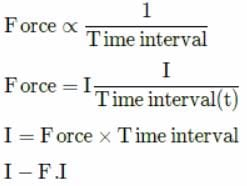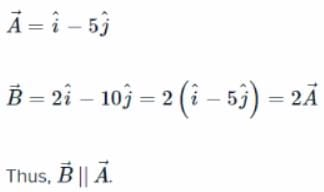NEET  >  Test: Scalars & Vectors (NCERT)

# Test: Scalars & Vectors (NCERT) - NEET

Test Description

## 5 Questions MCQ Test Physics Class 11 - Test: Scalars & Vectors (NCERT)

Test: Scalars & Vectors (NCERT) for NEET 2023 is part of Physics Class 11 preparation. The Test: Scalars & Vectors (NCERT) questions and answers have been prepared according to the NEET exam syllabus.The Test: Scalars & Vectors (NCERT) MCQs are made for NEET 2023 Exam. Find important definitions, questions, notes, meanings, examples, exercises, MCQs and online tests for Test: Scalars & Vectors (NCERT) below.
Solutions of Test: Scalars & Vectors (NCERT) questions in English are available as part of our Physics Class 11 for NEET & Test: Scalars & Vectors (NCERT) solutions in Hindi for Physics Class 11 course. Download more important topics, notes, lectures and mock test series for NEET Exam by signing up for free. Attempt Test: Scalars & Vectors (NCERT) | 5 questions in 5 minutes | Mock test for NEET preparation | Free important questions MCQ to study Physics Class 11 for NEET Exam | Download free PDF with solutions
 1 Crore+ students have signed up on EduRev. Have you?
Test: Scalars & Vectors (NCERT) - Question 1

### Which of the following is not a scalar quantity?

Detailed Solution for Test: Scalars & Vectors (NCERT) - Question 1

Impulse (I)Since force is a vector quantity and impulse is the product of the sudden force applied and the time interval to bring equivalent the system. So Impulse also a vector quantity which have same direction to the force applied.

Test: Scalars & Vectors (NCERT) - Question 2

### In Latin, the word vector means

Detailed Solution for Test: Scalars & Vectors (NCERT) - Question 2

In Latin, the word vector means carrier.

Test: Scalars & Vectors (NCERT) - Question 3

### A vector is not changed if

Detailed Solution for Test: Scalars & Vectors (NCERT) - Question 3

When a vector is displaced parallel to itself, neither its magnitude nor its direction changes.

Test: Scalars & Vectors (NCERT) - Question 4

Which of the following statements.is false regarding the vectors?

Detailed Solution for Test: Scalars & Vectors (NCERT) - Question 4

The magnitude of a vector is a pure number. Each component of a vector is also a vector. The resultant of two vectors can be zero only if they have the same magnitude and opposite directions.

Test: Scalars & Vectors (NCERT) - Question 5

Which of the following pairs of vectors are parallel?

Detailed Solution for Test: Scalars & Vectors (NCERT) - Question 5## Physics Class 11

130 videos|483 docs|210 tests
Information about Test: Scalars & Vectors (NCERT) Page
In this test you can find the Exam questions for Test: Scalars & Vectors (NCERT) solved & explained in the simplest way possible. Besides giving Questions and answers for Test: Scalars & Vectors (NCERT), EduRev gives you an ample number of Online tests for practice

## Physics Class 11

130 videos|483 docs|210 tests

### How to Prepare for NEET

Read our guide to prepare for NEET which is created by Toppers & the best Teachers(Scan QR code)